## Catmull-Rom splines¶

### Definition of this class of curves¶

The Catmull-Rom interpolation problem defined in [Catmull, E. and R. Rom, A Class of Local Interpolationg Splines, in Barnhill R.E. and R.F. Riesenfeld (eds.), Computer Aided Geometric Design, Academic Press, New York, 1974.] is formulated as follows:

Given $n+1$ points, $P_0, P_1, \ldots, P_n$, $n\geq 3$, find a piecewise cubic curve, parameterized by $c:[1, n-1]\to\mathbb{R}^d$ (usually, d=2,3) such that $c(i)=P_{i}$, for $i\in\{1,2\ldots, n-1\}$, and the tangent vector at each interpolatory point, $P_i$, $i=\overline{1, n-1}$, to be colinear with the vector $\overrightarrow{P_{i-1}P_{i+1}}$:

$$\vec{\dot{c}}(i)=s\overrightarrow{P_{i-1}P_{i+1}},$$

where $s\in(0,1]$. Usually one takes s=0.5.

Hence given n+1 points, $P_0, P_1, \ldots, P_n$, a CR curve interporlates only the points $P_1, P_2, \ldots, P_{n-1}$. The first and the last point influence only the direction of tangent at $P_1$, respectively, $P_{n-1}$.

Since the distance between two consecutive knots, $t_i=i$, $t_{i+1}=i+1$ is constant, such a curve is called uniform parameterized Catmull-Rom curve.

Being a cubic above each interval $[i, i+1]$, an arc of CR curve on such an interval can be defined as a Bézier curve of control points ${\bf b}_0, {\bf b}_1, {\bf b}_2, {\bf b}_3$, with ${\bf b}_0=P_i, {\bf b}_3=P_{i+1}$.

But the tangent at end points, ${\bf b}_0$ and ${\bf b}_3$, of a Bézier curve, is $3\overrightarrow{{\bf b}_0{\bf b}_1}$, respectively $3\overrightarrow{{\bf b}_2{\bf b}_3}$. Thus we get:

$${\bf b}_1=P_i+s(P_{i+1}-P_{i-1})/3, \:\: {\bf b}_2=P_{i+1}-s(P_{i+2}-P_{i})/3$$

Below we illustrate the above stated properties of a CR curve, defined by 7 points. Attached to the third arc, joining the points $P_3, P_4$, is the Bézier control polygon defining that arc of curve. The tangent at $P_2$ is obviously parallel to $\overrightarrow{P_1P_3}$.

In :
from IPython.display import Image
Image(filename='Imag/Catmull-Rom-curve.png')

Out: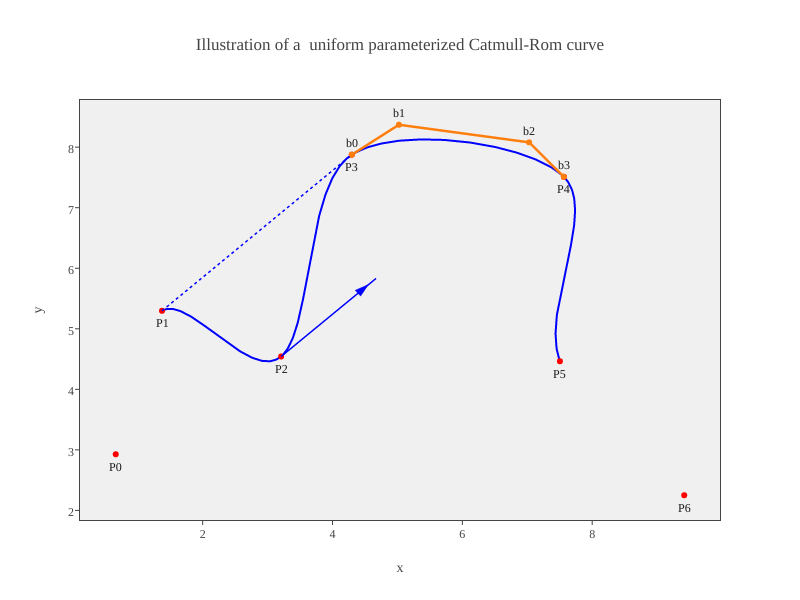Catmull-Rom interpolating curves can be evaluated at a point using a recursive scheme similar to the de Boor algorithm for B-spline curves.

Usually a cubic spline curve is $C^2$ at knots. Although the CR curves are only $C^1$ at each knot, they are sometimes called Catmull Rom spline curves, due to the similarities of the algorithms of evaluation, and other common properties.

Let us point out the drawback of the uniform parameterization, that led to defining CR splines with non-uniform knots.

A $C^1$-parameterization, $c:[a,b]\to\mathbb{R}^d$, of a curve can be interpreted as defining the motion of the point c(t), along the trace of $c$, during the time from a to b.

When the parameterization of a CR curve is uniform, the point $c(t)$ spends the same amount of time on each arc of ends $P_i, P_{i+1}$, $i=1,2, ...n-1$, irrespective of the distance between $P_i, P_{i+1}$.

If the distance between two data points is large, the point c(t) moves with a high speed. If the next two interpolatory points, $P_{i+1}, P_{i+2}$, are closer, then the moving point will overshoot since it cannot abruptly change its speed.

Barry and Goldman [P. J. Barry, R. N. Goldman, A recursive evaluation algorithm for a class of Catmull–Rom splines, SIGGRAPH Computer Graphics, 22(4):199-204, 1988] extended the definition of uniform parameterized CR splines to curves whose parameterization incorporates the distance between any two adjacent interpolatory points.

Namely, given the points $P_0, P_1, \ldots, P_n$, one defines a knot sequence, $t_0, t_1, \ldots, t_{n}$ as follows:

$$\begin{array}{lll}t_0&=&0\\ t_i&=&t_{i-1}+||\overrightarrow{P_{i-1}P_i}||^\alpha, \quad \alpha\in[0,1]\end{array}$$

A $C^1$-piecewise cubic, that interpolates the given points, i.e. $c(t_i)=P_i$, $i=1,2, \ldots, n-1$, is defined at any $u\in[t_i, t_{i+1}]$ through a recursive scheme in which are involved only four points, $P_{i-1}, P_i, P_{i+1}, P_{i+2}$ and the knots $t_{i-1}, t_i, t_{i+1}, t_{i+2}$:

$$P_j^r=(1-\omega^{r-1}_j)P^{r-1}_j+\omega^{r-1}_jP^{r-1}_{j+1}, \quad r=1,2,\quad j=i-1, i, i+1, i+2,$$

with $\omega^r_j(u)=\displaystyle\frac{u-t_j}{t_{j+1+r}-t_j}$. The superscript $r$ points out the level of recursion. $P_j^0=P_j$.

The last two points, $P^2_{i-1}$, $P^2_{i}$, are finally interpolated linearly, to get $c(u)$, the point on the CR curve corresponding to the parameter $u\in[t_i, t_{i+1}]$:

$$c(u)=\left(1-\displaystyle\frac{u-t_i}{t_{i+1}-t_i}\right) P^2_{i-1}+\displaystyle\frac{u-t_i}{t_{i+1}-t_i} P^2_{i}$$

We note that in the first two steps, the points $P_j^r$, $r=1,2$, are computed via de Boor algorithm for B-splines, while in the last step, $c(u)$ is computed according to the Neville algorithm for evaluating Lagrange polynomials.

The parameter $\alpha$ in the definition of knots controls the geometry of the interpolating CR curve. For $\alpha=0.5$ the corresponding CR curve is called centripetal Catmull-Rom curve, for $\alpha=1$, chordal Catmull Rom curve, while for $\alpha=0$ we get the initial uniform parameterized CR spline.

For $\alpha$ close to $0$ the corresponding CR curve can exhibit singular points (self intersections or cusps) within short curve segments(with small distance between $P_i, P_{i+1}$).

In [C Yuksel, S Schaefer, J Keyser, Parameterization and Applications of Catmull-Rom Curves, Computer Aided Design, 43, 7, 2011] it was deduced that the optimal parameter that corresponds to CR splines with no singular points is $\alpha=0.5$. Moreover a centripetal CR curve follows more tightly the interpolatory points than CR curves corresponding to $\alpha$ close to 0 or to 1.

That is why centripetal Catmull-Rom splines are now widely used in interactive generation of interpolating curves.

### Python implementation of the Catmull-Rom spline generation¶

Instead of the above mixture of the de Boor and Neville algorithm we generate each segment of CR curve, as a Bezier curve.

Over each interval $[t_i, t_{i+1}]$, the CR curve is a cubic polynomial curve, hence it can be defined as a Bézier curve, parameterized over $[0,1]$. Theoretically, the standard polynomial parameterization is obtained applying (using a Computer Algebra System) the two steps of de Boor algorithm defined above, and a step of the Neville algo. Then this parameterization is converted to one in which the polynomial components are expressed in Bernstein basis, to get the Bézier form of that polynomial segment of curve.

The expression of the Bézier control points as combinations of four consecutive interpolatory points, $P_{0}, P_1, P_{2}, P_{3}$, involved in the definition of a CR segment, is given in the function ctrl_bezier(P, d). d is a list of values involved in knot definition, $d_j=||\overrightarrow{P_{j}P_{j+1}}||^\alpha$, $j=0,1,2$.

In :
import numpy as np
import matplotlib.pyplot as plt
%matplotlib inline


Define the function knot_interval, that computes the values $d_j=||\overrightarrow{P_{j}P_{j+1}}||^\alpha$, $j=\overline{0, n-1}$. If we want define a closed curve, then we extend the list of points $P_0, P_1, \ldots, P_n$ with $P_0, P_1, P_2$:

In :
def knot_interval(i_pts, alpha=0.5, closed=False):
if len(i_pts)<4:
raise ValueError('CR-curves need at least 4 interpolatory points')
#i_pts is the list of interpolatory points P, P, ... P[n]
if closed:
i_pts+=[i_pts, i_pts, i_pts]
i_pts=np.array(i_pts)
dist=np.linalg.norm(i_pts[1:, :]-i_pts[:-1,:], axis=1)
return dist**alpha

def ctrl_bezier(P, d):
#Associate to 4 consecutive interpolatory points and the corresponding three d-values,
#the Bezier control points
if len(P)!=len(d)+1!=4:
raise ValueError('The list of points and knot intervals have inappropriate len ')
P=np.array(P)
bz=*4
bz=P
bz=(d**2*P-d**2*P +(2*d**2+3*d*d+d**2)*P)/(3*d*(d+d))
bz=(d**2*P-d**2*P +(2*d**2+3*d*d+d**2)*P)/(3*d*(d+d))
bz=P
return bz

def Bezier_curve(bz, nr=100):
#implements the de Casteljau algorithm to compute nr points on a Bezier curve

t=np.linspace(0,1, nr)
N=len(bz)
points=[]# the list of points to be computed on the Bezier curve
for i in range(nr):#for each parameter t[i] evaluate a point on the Bezier curve
#via De Casteljau algorithm
aa=np.copy(bz)
for r in range(1,N):
aa[:N-r,:]=(1-t[i])*aa[:N-r,:]+t[i]*aa[1:N-r+1,:]# convex combination
points.append(aa[0,:])
return points

def Catmull_Rom(i_pts, alpha=0.5,  closed=False):
#returns the list of points computed on the interpolating CR curve
#i_pts the list of interpolatory points P, P, ...P[n]
curve_pts=[]#the list of all points to be computed on the CR curve
d=knot_interval(i_pts, alpha=alpha, closed=closed)
for k in range(len(i_pts)-3):
cb=ctrl_bezier(i_pts[k:k+4], d[k:k+3])
curve_pts.extend(Bezier_curve(cb, nr=100))

return np.array(curve_pts)


Now we give a list of points and define the CR curve corresponding to $\alpha=0,0.5,1$:

In :
P=[[-0.72, -0.3], [0,0], [1., 0.8], [1.1, 0.5], [2.7, 1.2], [3.4, 0.27]]

curve0=Catmull_Rom(P, alpha=0, closed=False)
curve1=Catmull_Rom(P, alpha=0.5, closed=False)
curve2=Catmull_Rom(P, alpha=1.0, closed=False)
xp, yp=zip(*P)

fig=plt.figure(figsize=(9,6))
plt.plot(curve0[:,0], curve0[:,1], 'r', xp, yp, 'go' )
plt.plot(curve1[:,0], curve1[:,1], 'g')
plt.plot(curve2[:,0], curve2[:,1], 'b')

Out:
[<matplotlib.lines.Line2D at 0x7f75070e8b50>]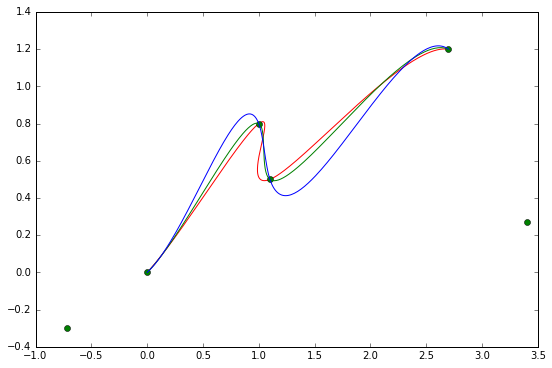The green curve is the centripetal CR spline interpolating the central 4 from 6 points. It folows closely the given interpolatory points.

In order to get more insights into CR curves properties we give the possibility to generate them interactively. Choose the interpolatory points, by clicking the left mouse button at the desired position. When the right button is pressed, the corresponding curve is generated:

In :
#enable interactivity in notebook:
%matplotlib notebook

In :
def curve_plot(i_pts,  alpha=0.5, closed=False):
curve_pts=Catmull_Rom(i_pts, alpha, closed=closed)
# plot the interpolating curve
plt.plot(curve_pts[:,0], curve_pts[:,1], 'b')

In :
class catrom(object):

def __init__(self,  alpha=0.5, closed=False):
self.interp_pts=[]     # list of interpolating points
self.alpha=alpha
self.closed=closed# boolean value
def callback(self, event):  #select interpolating points with  left  mouse button click
if event.button==1 and event.inaxes:
x,y = event.xdata, event.ydata
self.interp_pts.append([x,y])
plt.plot(x, y,  'bo')

elif event.button==3:   #press right  button to plot the curve
curve_plot(self.interp_pts,  self.alpha, self.closed)
plt.draw()

else: pass

def caxis(self):#define axes for plot
fig = plt.figure(figsize=(8, 6))

ax.set_xlim(0,10)
ax.set_ylim(0,10)
ax.grid('on')
ax.set_autoscale_on(False)
fig.canvas.mpl_connect('button_press_event', self.callback)

In :
curve=catrom()
curve.caxis()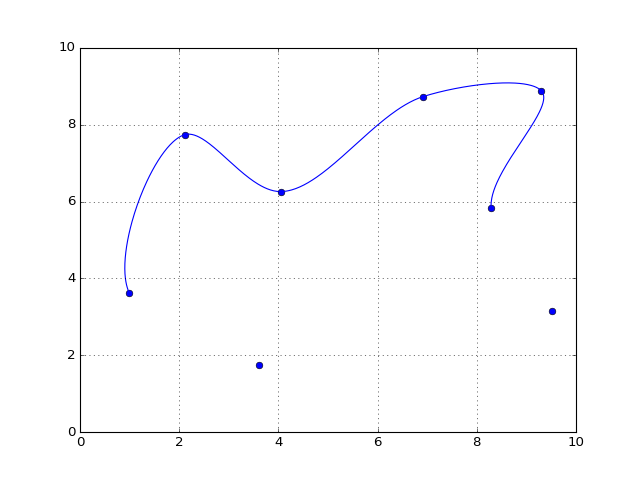In :
cv=catrom(closed=True)
cv.caxis()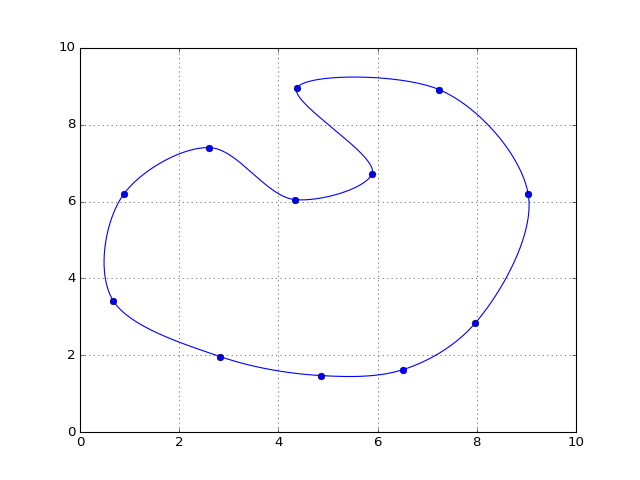In :
cv2=catrom(alpha=0)
cv2.caxis()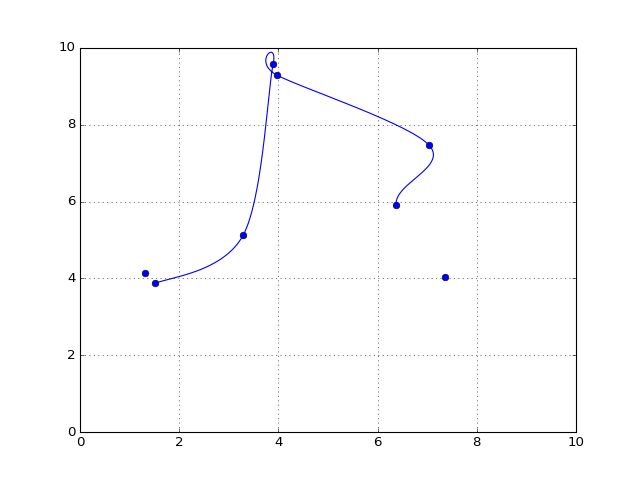The advantage of defining a segment of a CR curve as a Bezier curve, not via de Boor plus Neville algorithm, is that we can compute the tangent direction at any point of the curve.

Namely, if $r:[0,1]\to\mathbb{R}^d$ is the Bézier parameterization of a segment of CR curve, then the tangent vector at a point corresponding to the parameter $t\in[0,1]$ is $\vec{\dot{r}}(t)=3({\bf b}_1^{2}(t)-{\bf b}_0^2(t))$, where ${\bf b}^2_0, {\bf b}^2_1$ are points computed in the second level of recursion of the de Casteljau schema (for details see this notebook):

In :
def tangentBezier(bz,t):
#bz is the list of Bezier control points and t is value in [0,1]
if t<0 or t>1:
raise ValueError('The parameter t must bi in [0,1]')
a=np.copy(bz)
N=a.shape
for r in range(1,N-1):
a[:N-r,:]=(1-t)*a[:N-r,:]+t*a[1:N-r+1,:]

return (len(bz)-1)*(a[1,:]-a[0,:])



The above functions are independent on the dimension of the space of points. They work well on points in any space $\mathbb{R}^d$, $d\geq 2$.

Here is an illustration of a Plotly plot of a (2,3)-torus knot resulted from a centripetal Catmull-Rom interpolation of a set of points on torus:

In :
from IPython.display import HTML
HTML('<iframe src=https://plot.ly/~empet/13914/ width=900 height=600></iframe>')

Out:
In :
from IPython.core.display import HTML
def  css_styling():